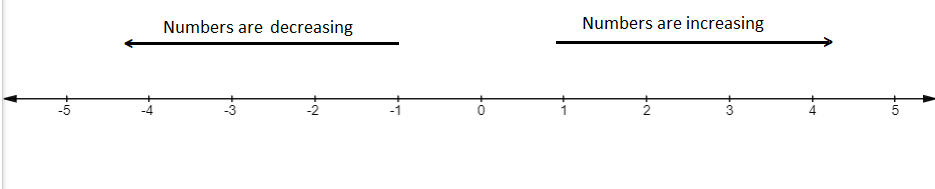Courses
Courses for Kids
Free study material
Free LIVE classes
MoreLIVE
Join Vedantu’s FREE Mastercalss

# Arrange the following in ascending and descending order with the help of number line:(-2), 0, 3, (-5)Verified
269.8k+ views
Hint: In this question we have to find out the ascending and descending order of the numbers. Think upon this question by making a number line and analyze it.

We have been given numbers (-2), 0, 3, (-5) and we have to find the ascending and descending order of the same.
So, we will start the question by making a number line.
First, we will draw a horizontal line then we will mark zero on the center of the line then we will mark other numbers at equal intervals.As, we can see in the number line the numbers moving towards the left side are decreasing and the numbers moving towards the right side are increasing.
Now, we know that in ascending order the numbers are increasing and in descending order numbers are decreasing.
So, the ascending order of the numbers is,
(-5), (-2), 0, 3
And, the descending order of the numbers is,
3, 0, (-2), (-5)

Note: Whenever we face such types of problems the key point to remember is that we need to have a good grasp over the number line, like one drawn above. A straight line with numbers placed at equal intervals or segments along its length is called a number line. A number line can be extended infinitely in any direction and is usually represented horizontally.
Last updated date: 16th Sep 2023
Total views: 269.8k
Views today: 6.69k